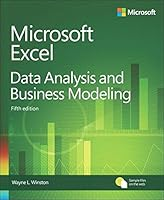# Microsoft Excel Data Analysis and Business Modeling, 5th Edition0 Reviews
2016-12-24
864 pages

## Book Description

Master business modeling and analysis techniques with Excel 2016, and transform data into bottom-line results. Written by award-winning educator Wayne Winston, this hands on, scenario-focused guide helps you use Excel’s newest tools to ask the right questions and get accurate, actionable answers. This edition adds 150+ new problems with solutions, plus a chapter of basic spreadsheet models to make sure you’re fully up to speed.

• Quickly transition from Excel basics to sophisticated analytics
• Summarize data by using PivotTables and Descriptive Statistics
• Use Excel trend curves, multiple regression, and exponential smoothing
• Master advanced functions such as OFFSET and INDIRECT
• Delve into key financial, statistical, and time functions
• Leverage the new charts in Excel 2016 (including box and whisker and waterfall charts)
• Make charts more effective by using Power View
• Tame complex optimizations by using Excel Solver
• Run Monte Carlo simulations on stock prices and bidding models
• Work with the AGGREGATE function and table slicers
• Create PivotTables from data in different worksheets or workbooks
• Learn about basic probability and Bayes’ Theorem
• Automate repetitive tasks by using macros

Chapter 2 Range names
Chapter 3 Lookup functions
Chapter 4 The INDEX function
Chapter 5 The MATCH function
Chapter 6 Text functions
Chapter 7 Dates and date functions
Chapter 8 Evaluating investments by using net present value criteria
Chapter 9 Internal rate of return
Chapter 10 More Excel financial functions
Chapter 11 Circular references
Chapter 12 IF statements
Chapter 13 Time and time functions
Chapter 14 The Paste Special command
Chapter 15 Three-dimensional formulas and hyperlinks
Chapter 16 The auditing tool
Chapter 17 Sensitivity analysis with data tables
Chapter 18 The Goal Seek command
Chapter 19 Using the Scenario Manager for sensitivity analysis
Chapter 20 The COUNTIF, COUNTIFS, COUNT, COUNTA, and COUNTBLANK functions
Chapter 21 The SUMIF, AVERAGEIF, SUMIFS, and AVERAGEIFS functions
Chapter 22 The OFFSET function
Chapter 23 The INDIRECT function
Chapter 24 Conditional formatting
Chapter 25 Sorting in Excel
Chapter 26 Tables
Chapter 27 Spin buttons, scroll bars, option buttons, check boxes, combo boxes, and group list boxes
Chapter 28 The analytics revolution
Chapter 29 An introduction to optimization with Excel Solver
Chapter 30 Using Solver to determine the optimal product mix
Chapter 31 Using Solver to schedule your workforce
Chapter 32 Using Solver to solve transportation or problems
Chapter 33 Using Solver for capital budgeting
Chapter 34 Using Solver for financial planning
Chapter 35 Using Solver to rate sports teams
Chapter 36 Warehouse location and the GRG Multistart and Evolutionary Solver engines
Chapter 37 Penalties and the Evolutionary Solver
Chapter 38 The traveling salesperson problem
Chapter 39 Importing data from a text file or
Chapter 40 Validating data
Chapter 41 Summarizing data by using histograms and Pareto charts
Chapter 42 Summarizing data by using descriptive statistics
Chapter 43 Using PivotTables and slicers to describe data
Chapter 44 The Data Model
Chapter 45 Power Pivot
Chapter 46 Power View and Maps
Chapter 47 Sparklines
Chapter 48 Summarizing data with statistical functions
Chapter 49 Filtering data and removing duplicates
Chapter 50 Consolidating data
Chapter 51 Creating subtotals
Chapter 52 Charting tricks
Chapter 53 Estimating straight-line relationships
Chapter 54 Modeling exponential growth
Chapter 55 The power curve
Chapter 56 Using correlations to summarize relationships
Chapter 57 Introduction to multiple regression
Chapter 58 Incorporating qualitative factors into multiple regression
Chapter 59 Modeling nonlinearities and interactions
Chapter 60 Analysis of variance: One-way ANOVA
Chapter 61 Randomized blocks and two-way ANOVA
Chapter 62 Using moving averages to understand time series
Chapter 63 Winters method
Chapter 64 Ratio-to-moving-average forecast method
Chapter 65 Forecasting in the presence of special events
Chapter 66 An introduction to probability
Chapter 67 An introduction to random variables
Chapter 68 The binomial, hypergeometric, and negative binomial random variables
Chapter 69 The Poisson and exponential random variable
Chapter 70 The normal random variable and Z-scores
Chapter 71 Weibull and beta distributions: Modeling machine life and duration of a project
Chapter 72 Making probability statements from forecasts
Chapter 73 Using the lognormal random variable to model stock prices
Chapter 74 Introduction to Monte Carlo simulation
Chapter 75 Calculating an optimal bid
Chapter 76 Simulating stock prices and asset-allocation modeling
Chapter 77 Fun and games: Simulating gambling and sporting- probabilities
Chapter 78 Using resampling to analyze data
Chapter 79 Pricing stock options
Chapter 80 Determining customer value
Chapter 81 The economic order quantity inventory model
Chapter 82 Inventory modeling with uncertain demand
Chapter 83 Queuing theory: The mathematics of waiting in line
Chapter 84 Estimating a demand curve
Chapter 85 Pricing products by using tie-ins
Chapter 86 Pricing products by using subjectively determined demand
Chapter 87 Nonlinear pricing
Chapter 88 Array formulas and functions
Chapter 89 Recording macros

## Book Details

• Title: Microsoft Excel Data Analysis and Business Modeling, 5th Edition
• Author:
• Length: 864 pages
• Edition: 5
• Language: English
• Publisher:
• Publication Date: 2016-12-24
• ISBN-10: 1509304215
• ISBN-13: 9781509304219